# The Living Algorithm

## Introduction

An Open Recursive Function

Produces Data Stream Derivatives

A Nested Function

Interlocking Systems of Dynamics & Probability

Rough Approximations, not Precise Predictions

Attention entrains to LA's Mathematical Processes

Mathematical Processes associated with Number Strings

Number String Interruptions: Mathematical Behavior

Essence vs. Process: Content vs. Context

Living Algorithm Features: Table

### Synopsis

This page provides an introduction to the Living Algorithm, the mathematical foundation for my Theory of Attention. The LA's sole purpose is to digest data streams, i.e. transform raw data into a form that is useful to living systems. In contrast to the closed, set-based mathematics of Matter, the LA is both open to external data and based in reflexive, feedback loops. By applying the algorithm to its results, i.e. digested data streams, the LA produces information that would be useful to any living system, for instance the ongoing dynamics and probabilities associated with any data stream, both numerical and relative. These ongoing data streams are the foundation for DSD, the mathematical system that is the heart of our Theory of Attention.

I am proud to present the Living Algorithm (LA), the mathematical foundation for our Theory of Attention. What does this mean?

A lifetime of personal research coalesced in a mathematically-based theory regarding the rhythms of Attention. The Math-Data synergy associated with the theory indicates that Attention follows some distinct rules. For instance, Attention exhibits Pulse-like behavior.The mathematics behind the theory is based solely upon the Living Algorithm. There are many patterns of correspondence between the mathematical processes of this algorithm and experimentally verified phenomenon associated with living systems, especially attention. These patterns are not limited to a single area of focus, but instead extend to both our behavior and our biological systems, including neural networks. The author’s Attention Theory was developed to make sense of these many parallels.The theory is based around the notion that the Living Algorithm provides the point-of-contact mathematical interface between human attention and environmental information. In other words, our Attention Network digests information via this algorithm1 .### Living Algorithm: an Open Recursive Function

The Living Algorithm is a deceptively simple equation that generates an amazingly complex mathematical system. Following is the most basic form of the Living Algorithm. It computes a type of running average for the most recent moment in a data stream.Applying this simple algorithm to itself yields a multitude of fascinating mathematical features. These include an ongoing, interlocking system of probability and dynamics. More startling still, human attention tends to become entrained to the processes of the Living Algorithm – hence its name.

What unique features does the Living Algorithm possess that provides it with these special abilities?

The Living Algorithm is an open recursive function.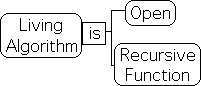What does this mean? Recursive functions are both reflexive, i.e. self-referential, and iterative, i.e. the repetition of a set process.The Living Algorithm is also open. With each iteration/repetition of the process, new information enters the system. As such, the results are dependent upon the incoming flow of data, i.e. the data stream. With each iteration, the initial conditions fade in significance. As such, the current data is much more significant than the initial conditions in terms of results.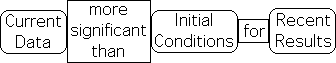Traditional mathematical functions are fundamentally different. Instead of being open, they are closed to new information. As such, the initial conditions are of utmost importance. Also, they are certainly not self-referential.

### Living Algorithm produces Data Stream Derivatives

The Living Algorithm (LA) specializes in data streams - an ongoing flow of information. The LA’s sole purpose is to operate upon, i.e. digest1a, data streams. The digestion process yields the rates of change, i.e. derivatives, of the data stream.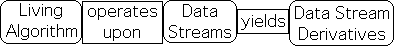These data stream derivatives only apply to the most recent data point in the data stream, not the entire set. The derivatives characterize both the trajectory and probabilistic tendencies of data stream's most recent position. In contrast to the data point, i.e. the instant, these measures provide dynamic context to what has been deemed the current moment. As such, it could be said that the Living Algorithm computes the mathematics of the moment.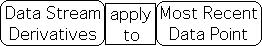### Living Algorithm: a Nested Function

How is the Living Algorithm able to generate a series of derivatives? As a recursive function, it is self-referential in that the current result is a function of the previous result. The algorithm can also be applied to itself. In this sense, Living Algorithm can act as a nested function. As a nested function, the Living Algorithm is able to generate the higher derivatives.To better understand the concept of a nested function, let us look at the mathematics. The simplest form of the Living Algorithm shown above can be written in the following form. The data stream's current velocity/average is a function of the current point in the data stream XN and the previous average.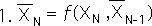Applying the Living Algorithm to itself, we get an expression for the data stream's acceleration. The 2 superscript indicates acceleration. This process can be repeated indefinitely to get the higher derivatives with superscripts of 3, 4 and so forth. The current acceleration is a function of the difference between the current point in the data stream and the past velocity, and the past acceleration.The past velocity is a function of the past data point and the velocity just before.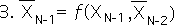The following expression is the result when expression 3 is substituted into expression 2. In other words, the acceleration is a function of a function. With each higher derivative, another self-referential function is added.### Living Algorithm generates Interlocking Systems of Dynamics & Probability

With these nested and self-referential features, the Living Algorithm's computational process generates a complex interlocking system of dynamics and probability – deemed Data Stream Dynamics (DSD).Data Stream Dynamics is based upon the constructs of Newtonian mechanics, including the notions of data stream acceleration, force and power. DSD seems to provide a mathematical framework for the natural rhythms of Attention that permeate our day-to-day lives. Human attention, in particular, appears to be entrained to the mathematical rhythms of DSD.

The probabilistic system includes averages, deviations and even correlations. As these measures only apply to the most recent data point, they could be deemed a type of running measure, for instance a running average. These measures provide invaluable information regarding the trajectories of the data stream.

The velocity of the data stream is also its running average, which also indicates the probable location of the next data point in the stream.The acceleration of the data stream, as computed by the Living Algorithm, has 2 components - a scalar and a directed component. The scalar component of the data stream's acceleration also represents the Deviation of the data stream. It indicates the probable range of the next data point. The directed component has been deemed the Directional of the data stream. It indicates the probable tendencies of the next data point.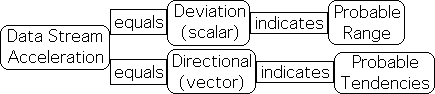### Rough Approximations, not Precise Predictions

The Living Algorithm generates a new trio of data stream derivatives with each iteration of the mathematical process. Each trio is unique in that it only applies to the current moment, the Nth position, not the entire data stream. The trio of measures describes the probabilistic trajectories of the most recent moment to provide dynamic context.

Because the trio describes the dynamics of the current moment, these data stream derivatives also predict the location of the next point in the data stream. Because of these features, the derivatives act as predictive descriptors. Due to the ever-changing dynamic nature of the derivatives combined with their predictive capacity, the trio as a group is called the Predictive Cloud.

Due to its open nature, fresh information enters the system with each iteration of the digestion process. This new information has a significant impact upon the results, as it is immediately incorporated into the trio of data stream derivatives, i.e. the Predictive Cloud.

A living data stream has a high degree of fluctuation. Indeed, one of the LA’s primary functions is to provide some central measures that approximate the order behind the inherently erratic nature of the data. However, these are rough approximations rather than precise predictions. Because of this lack of precision, the ability of these predictive descriptors to predict the future is limited. Although the predictions lack precision, these analytics are better than nothing, We suggest that the LA’s rough analytics could readily enable living systems to better deal with the erratic nature of existence.2### Attention entrains to LA's Mathematical Processes

If the predictive descriptors only provide rough approximations, what is the scientific relevance of the Living Algorithm's computational process?

While unable to make precise predictions regarding the future, the mathematical processes of the Living Algorithm seem to be highly significant for human beings. These innate processes appear to guide and shape our existence. Adhering to these innate processes enhances both our cognitive and physical performance. Conversely, ignoring them is detrimental, even destructive, to our continuing ability to fulfill potentials. Further, both our behavior and biology, especially associated with attention, tend to entrain to these mathematical processes. The scientific utility is based in this synchronization of mathematical process with the processes of both our intentional and physical existence.### Mathematical Processes associated with Number Strings

Which of the Living Algorithm's mathematical processes are most significant in terms of human entrainment?

Probably the LA's most fascinating mathematical feature concerns the behavior/processes associated with the data stream derivatives of a Number String. A Number String by definition is a data stream composed of identical elements. These identical elements can be numerical or non-numerical. For example, the identical elements could be the number 4, the color blue, or even your mother. Let us call the value/content of these identical elements, the 'essence' of the Number String.

When the LA digests a Number String, the essence does not enter into the computations. The essence can be factored out of the data stream. This leaves a Number String consisting solely of 1s - the Basic Number String.

As mentioned, the sole purpose of the Living Algorithm is to generate the successive derivatives of a data stream. The most fundamental of these is the data stream's velocity. The LA also generates the data stream's acceleration. As with Physics, the acceleration is by far the more significant of the two.

The Pulse of Acceleration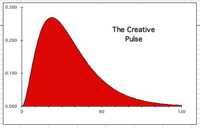When the Living Algorithm digests the Basic Number String, i.e. a data stream composed solely of 1s, the data stream's acceleration describes a pulse that begins, peaks and then fades out. The data stream acceleration behaves in the same manner no matter which Number String is digested. Further, the essence of the Number String can be numerical or non-numerical. This Pulse of Acceleration is shown at right.

The Pulse is the primary mathematical process of the Living Algorithm that is associated with human behavior. Human behavior, in particular, exhibits a pulse-like nature. In other words, our experiences have a relatively distinct duration, complete with beginning, peak and end. This pulsing extends to brain waves, attention span, music and even the sleep-wake cycle. Further the behavior of our pulses of experience exhibit distinct parallels with the mathematical behavior of Number String derivatives. For instance, these parallels apply to both interruptions and the necessity of down time.

### Number String Interruptions: Mathematical Behavior

Let us review some of these mathematical 'behaviors' of the Number String's acceleration. If a Number String of identical elements is 'interrupted' by the introduction of a non-identical element, the ideal potentials of the Pulse are diminished. We 'interrupted' the Number String of 1s with some '0's at a variety of points in the stream. A graphic visualization of the results is shown below. The ideal Pulse is indicated by the 'red' pulse in the background. The 'interrupted' Pulses are overlaid in the foreground.

The ideal Pulse (red) w/InterruptionsYet another feature of the Pulse bears mentioning. The Pulse fades out permanently, as long as the Number String continues. The only way to 'refresh' this Pulse is to follow the first Number String with a Number String that has a different essence. The ensuing Number String must be of sufficient duration to completely refresh the first. The graph at right is a visualization of the acceleration of a data stream consisting of a Number String of 1's followed by a Number String of 0's, and then a Number String of 1's. This intriguing data stream acceleration has been deemed the Triple Pulse.

As mentioned, the 'refreshing' Number String must be of sufficient duration. When the middle Number String of 0's, deemed the Empty Number String, is shortened, this has a negative impact upon the ideal dimensions of the final Pulse (here called the Active Pulse). Following is a visualization of the data stream acceleration, when the middle Number String of 0's is shortened, i.e. the final Active Pulse gets a 'headstart'.

Negative Impact of Shortened Middle Pulse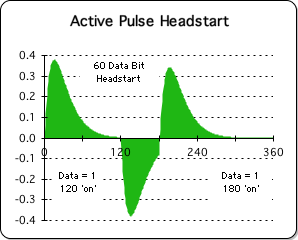Strangely enough, 'interruptions' to the middle Number String of 0's also has a negative impact upon the ideal dimensions of the final Pulse. Following is a visualization of the data stream acceleration when the middle Number String of 0's (here called the Rest Pulse) is interrupted by 1's.### Essence vs. Process: Content vs. Context

A highly significant feature of the Living Algorithm's digestion process concerns the dichotomy between Content vs. Context and the related topic of Essence vs. Process. The essence of the Number String remains the same throughout the process. However the impact of the essence is dramatically different depending upon the context. Let us provide some examples.

Let us consider the Basic Number String, composed solely of 1s. As mentioned, the acceleration describes a Pulse. The 1's at the beginning of the Pulse drive the acceleration upwards, while the 1s at the end of the Pulse have virtually no impact at all. Context is more important than content in terms of impact upon the acceleration.

The Living Algorithm digests the same Number String of 1s to generate both the 1st and 3rd Pulse of the Triple Pulse. However, when the middle 'Rest' Pulse is 'shortened' or 'interrupted', the dimensions of the final Pulse are diminished. In other words, the composition of the middle Number String of 0s exerts a significant impact upon the dimensions of the final Pulse. Only when the middle Number String is both uninterrupted and of sufficient duration does the final Pulse achieve its maximum proportions. In this case, the dynamic context of the mathematical process is more important than the Number String's essence in determining the proportions of the Pulse of Acceleration.Human attention, in particular, seems to be entrained to these mathematical processes. Despite our relatively fixed biological energy, our attention span is finite with distinct limits. The negative impact of interruptions upon our attention is disproportionate to their duration. And downtime is necessary to refresh our attention, as epitomized by our daily sleep-wake cycle. The confluence of patterns of correspondence between these differing types of human behavior and the mathematical processes of the Living Algorithm led to the Physics of Information accompanied by our Theory of Attention.

### Living Algorithm Features: Table

Summarizing for retention. As opposed to traditional equations, the Living Algorithm is an open recursive function. Its sole purpose is to compute the ongoing derivatives of any data stream. These derivatives establish the dynamic context of the data stream's current moment. As a nested function, the Living Algorithm also generates a system of both information dynamics and probability. However, the real significance of the Living Algorithm lies in the mathematical processes associated with Number Strings, data streams composed of identical elements. Human attention, in particular, tends to become entrained to these processes.

Below is a table that lists the special features of the Living Algorithm. I have developed the actual mathematics behind the Living Algorithm, i.e. the derivations and discussions, in a variety of articles and Notebooks, i.e. article streams.

Special Features of the Living Algorithm
Self Referential (Reflexive) Function
Iterative (Based in Repetition of a Process)
Open to External Information
Sole Purpose: Digesting Data Streams
Mathematical Process reveals Data Stream Derivatives (Moment's Dynamic Context)
Nested Function (Operates upon Itself as a Function)
Generates Interlocking System of Dynamics & Probability
Descriptive Predictors make Rough Approximations, not Precise Predictions
Data Stream Acceleration of Number String (identical elements) describes a Pulse
Interruptions diminish Ideal Proportion of the Pulse
New Number String (different essence) required to refresh Pulse
If New Number String is too short or interrupted, Ideal Dimensions of Refreshed Pulse diminished.
Dynamic Context of Mathematical Process more important than Numerical Essence

To see which of these features is unique to the Living Algorithm, check out the article Differences from Traditional Mathematics.

### Footnotes

1 For a more detailed analysis, check out the article – Attention's Mathematical Tool.

1a Rather than the more traditional word 'process', I employ the word 'digest' to indicate the Living Algorithm's computational process. The reasons are twofold. First, Infodynamics theory links food digestion with the LA's data digestion process. One provides nourishment for the Body; the other for the Mind. A secondary intent is to differentiate the LA's computations from standard electronic processing. While electronic processing frequently aims at exact replication, whether text, music or video, the LA's digestion process generates composite figures that establish dynamic context. Although incorporated into these composite measures, the raw data is lost.

2 If we but consider weather prediction, we can gain some appreciation for the value of these approximations regarding the future. Although the predictions provided by weather networks are frequently wrong, most of us attend the information, as it is also frequently right.